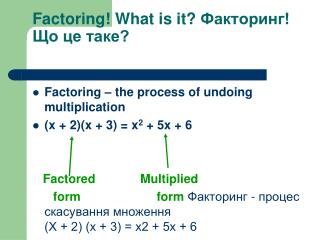DownloadDownload PresentationFactoring! What is it? Факторинг! Що це таке?

# Factoring! What is it? Факторинг! Що це таке?

Télécharger la présentation## Factoring! What is it? Факторинг! Що це таке?

- - - - - - - - - - - - - - - - - - - - - - - - - - - E N D - - - - - - - - - - - - - - - - - - - - - - - - - - -
##### Presentation Transcript

1. Factoring! What is it? Факторинг! Що це таке? • Factoring – the process of undoing multiplication • (x + 2)(x + 3) = x2 + 5x + 6 Factored Multiplied form formФакторинг - процес скасування множення(Х + 2) (х + 3) = x2 + 5x + 6

2. Factoring • x(x – 6) = x2 – 6x Factored Multiplied form form

3. How do we factor? • Let’s refer to the graphic organizer. • We will start at the top. • Звернемося до графічних організатором.Почнемо зверху First: Find and remove the GCF (greatest common factor)

4. How to Find the Greatest Common Factor (GCF • The greatest common factor of two or more whole numbers is the largest whole number that divides evenly into each of the numbers.

5. How do we find the GCF of variables? Choose the variable with the smallest exponent. • What is the GCF of x and x2? • What is the GCF of x3 and x? • What is the GCF of x2 and x3?

6. What is the GCF? • 3x – 6 • 2x + 12 • 12x + 9 • x2 – 6x • 4x2 – 2x • 5x3 – 15x2

7. Now let’s FACTOR by finding and removing the GCF! • Remove GCF and in parentheses write what is left • 3x – 6 GCF = 3 • 3( )What is left after 3 is removed? • 3(x – 2) Answer

8. How do we factor out this problem..

9. Factor – You Try! • 3x – 6 • 2x + 12 • 12x + 9 • x2 – 8x • 4x2 – 12x • 5x3 – 25x2

10. Factoring by GroupingLook at the graphic organizer! First: Find and remove the GCF How many terms does the polynomial have? 4 Use factor by grouping method

11. Let’s look at our graphic organizer GCF Find and remove the GCF How many terms does the polynomial have? trinomial 3 Use trial and error method of factoring.

12. Now let’s FACTOR TRINOMIALS!3 terms • Remember, we undo multiplying! • x2 + 5x + 6 1. Is there a GCF? • ( x + 2 )( x + 3 ) To factor a trinomial, it breaks down into a product of binomials

13. Factoring Trinomials • x2 + 5x + 6 ( x ) ( x ) x2 = x ▪ x • What are the factors of 6? 1, 6 -1, -6 2, 3 -2, -3 • Which pair adds to be 5? 2, 3 • (x + 2)(x + 3) Answer

14. Factor Trinomials – You Try! • x2 + 7x + 12 • x2 + 12x + 20 • x2 – 2x – 24 • x2 + 8x + 12 • x2 – x – 12 • x2 – 6x + 8 • x2 – 11x + 24 • x2 + 6x + 9

15. Let’s look at our graphic organizer GCF Find and remove the GCF binomial How many terms does the polynomial have? 2 Difference of Two Squares

16. What’s a Difference of Two Squares • Must have 2 perfect squares • Must have subtraction (difference) • A variable is a perfect square if the exponent is an even number.

17. Differences of Two Squares • IS IT A DTS? • X2 + 25 • X2 – 16 • X5 – 81 • 16x2 – 100 • 25x4 – 16x • X2 + 10x + 25

18. Factor. Use graphic organizer. 1. x2 – 16 2. x2 – 100